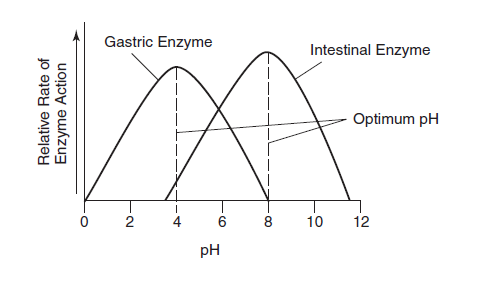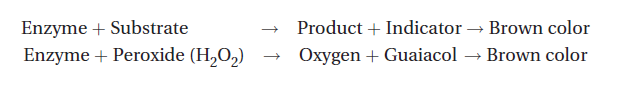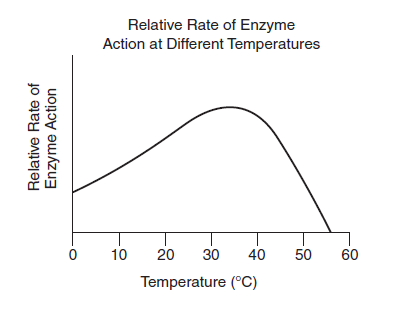# AP Biology Long Free-Response Questions

###### August 1, 2021Section II of the AP Biology exam consists of two long free-response questions and four short free-response questions. The two long free-response questions take about 25 minutes each to answer, are usually worth 8 to 10 points each, focus on interpreting and evaluating experimental results (with and without graphing), and are broken down into four parts each.

## Sample AP Biology Long Free-Response Question

(Note: This question is worth 10 points total.)

• (a) Describe and explain what an enzyme is and how it functions. (2 points)
• (b) Choose one enzyme, and identify experimental design procedures (using different conditions of temperature or pH) that would demonstrate inhibition of an enzymatic reaction. (4 points)
• (c) Below is a graph that shows the rate of two enzyme-mediated reactions. Analyze the results shown on this graph. (2 points)
• (d) Make your own predictions about the results that you would expect for the experiment you designed in part (b). Justify your predictions. (2 points)## Sample AP Biology Long Free-Response Answer

(a) An enzyme is a protein catalyst that reacts with every substrate and speeds up every type of reaction. An enzyme speeds up reactions by lowering the reaction’s energy of activation (Eₐ), which is the amount of energy that is required to start the reaction. Enzymes are specific, meaning they only react in certain ways with certain substrates. This specificity is determined by the shape of the substrate and the shape (conformation) of the enzyme. The specific shape of an enzyme results from interactions among the R-groups of the amino acids that make up the enzyme. In order for the substrate to bond to an enzyme, the substrate slightly alters the shape of an enzyme, so that the fit is exact. The model for the specificity of enzyme function is called “lock and key.”

(b) I would choose an enzyme that was recently extracted from the human liver. The easiest way to measure the amount of oxygen that is released is to use an indicator called guaiacol, which turns a brownish color when it binds to oxygen in a solution. One can determine how much oxygen is in a sample simply by observing how brown the solution is.

I would make a series of dilutions of guaiacol, plus different amounts of oxygen, that range from clear (little oxygen present) to dark brown (lots of oxygen present). I would then use a spectrophotometer to quantify how dark the color of the dilution is, which will help me then determine the amount of oxygen that is present in each tube. Then, I would use the guaiacol dilutions for comparison once I had results from the experiment.

The reaction that is the basis of this experiment is as follows:To determine the rate of enzyme activity and the inhibition of an enzymatic reaction, I would carry out this experiment using the following different temperatures: 4°C, 15°C, 25°C, 38°C, 50°C, 70°C, and 100°C.

(c) The graph shows two enzyme-mediated reactions. One of these reactions shows an enzyme that was extracted from the stomach, an environment that is acidic. The other reaction shows an enzyme that was extracted from the intestine, an environment that is normally basic (alkaline).

The reaction with the gastric enzyme shows no reactivity at pH 0, with activity increasing in the acidic range until it reaches its maximum rate (velocity) of activity (Vmax) at the optimum pH 4. Above pH 4, the reaction slows down, decreasing to zero by pH 8.

The reaction with the intestinal enzyme shows no reactivity at approximately pH 3.8, with increasing activity until it reaches its maximum rate (Vmax) at its optimum pH 8. Above pH 8, the reaction slows down, eventually reaching zero by pH 11.8.

(d) In the experiment from part (b), I predict that the rate of the enzyme-controlled reaction would increase from zero to its maximum rate (at the optimal temperature [body temperature] of approximately 38°C), and then the reaction would be inhibited and the rate of the reaction would decrease until the reaction ceased totally. Refer to the graph below.I made this prediction because each enzyme functions in the way that it does because of its unique shape or conformation. The rate of an enzyme reaction is optimal within a narrow range of temperatures. Outside that narrow range, the intramolecular bonds that hold an enzyme in its specific conformation break, causing the enzyme to lose its special shape. Enzymes denature at high temperatures, and, when they do, the reaction is inhibited.

## Analysis of Sample AP Biology Long Free-Response Answer

Although the student may have presented many correct statements in each part of his or her responses, remember that it is only possible to earn the maximum number of points that are stated for each part of the question. For example, even if the student included 8 correct statements in his or her response to part (a), the student could only receive the maximum number of points for that part, which in this case is 2 points. If a term was used but not defined or explained correctly, no credit was awarded. If the student contradicted himself or herself within an answer to one part of the question, the student lost all points for that part of the question.

Out of 10 total points possible, this answer would have earned 6 points. The breakdown of these point distributions is as follows:

#### Answer Part (a)

The student received points for correctly stating the following:

• Enzymes lower a reaction’s energy of activation (Eₐ), which is the amount of energy that is required to start the reaction. (1 point)
• Enzymes are specific because of their shape or conformation. (1 point)
• The specific shape of an enzyme results from interactions among the R-groups of the amino acids that make up the enzyme. (1 point)

The student also included some contradictory statements and/or incorrect statements and/or was missing information (for which he or she either lost points [only for the contradictory statements] or did not earn any points):

• An enzyme is a protein catalyst that reacts with every substrate and speeds up every type of reaction. (Not only is this statement incorrect, but it also contradicts the statement in the third sentence of the sample answer, which is correct and states that “Enzymes are specific, meaning they only react in certain ways with certain substrates.”)
• The model for the specificity of enzyme function is called “lock and key.” (The correct name for the model is “induced fit.” However, the student would not lose points for stating incorrect information; the student would only lose points for contradicting himself or herself.)

In this case, the student made a contradictory statement, so he or she would have lost all points for part (a) of this question. Thus, the student received 0 points out of a possible 2 points total for part (a)

#### Answer Part (b)

The student received points for correctly stating the following:

• The easiest way to measure the amount of oxygen that is released is to use an indicator called guaiacol, which turns a brownish color when it binds to oxygen in a solution. (1 point)
• I would then use a spectrophotometer to quantify how dark the color of the dilution is, which will help me then determine the amount of oxygen that is present in each tube. (1 point)
• To determine the rate of enzyme activity and the inhibition of an enzymatic reaction, I would carry out this experiment using the following different temperatures: 4°C, 15°C, 25°C, 38°C, 50°C, 70°C, and 100°C. (1 point)

The student also included some incorrect statements and/or was missing information (for which he or she did not earn any points):

• The student did not name the enzyme that was used. (Peroxidase is an enzyme, found in all cells, that breaks down peroxide, which is a toxic by-product of cellular metabolism.)
• The student did not explain how the spectrophotometer measures color by the rate of absorbance or transmittance of light. (If the spectrophotometer is set to measure absorbency, then the darker brown the sample is, the more light will be absorbed by the sample and the more oxygen it will contain.)

Thus, the student received 3 points out of a possible 4 points total for part (b)

#### Answer Part (c)

The student received a point for correctly stating the following:

• The student correctly identified which enzymes are shown on the graph and correctly identified the arc of each enzyme reaction. (1 point)
• The student also included some incorrect statements and/or was missing information (for which he or she did not earn any points):
• The student did not describe the graph perfectly. (The student did not specifically state the labels on the x-axis and the y-axis. Remember, when you draw a graph or describe a graph on the actual exam, your drawing or description must be complete, including the labels for all axes.)

Thus, the student received 1 point out of a possible 2 points total for part (c).

#### Answer Part (d)

The student received points for correctly stating the following:

• The student correctly made a prediction about the results that he or she would expect for the experiment designed in part (b). The prediction was that the enzyme-­controlled reaction would be inhibited above approximately 38°C (body temperature) and that the decrease in the rate of the reaction would continue until the reaction ceased totally. (1 point)
• The student also correctly justified his or her prediction. The student correctly explained the cause of denaturation in an enzyme (that heat breaks intramolecular bonds that give the enzyme its specific shape and function). The student also stated that denaturation inhibits an enzyme-controlled reaction. (1 point)

Nothing was incorrect or missing from this response. Thus, the student received 2 points out of a possible 2 points total for part (d).

SHARE